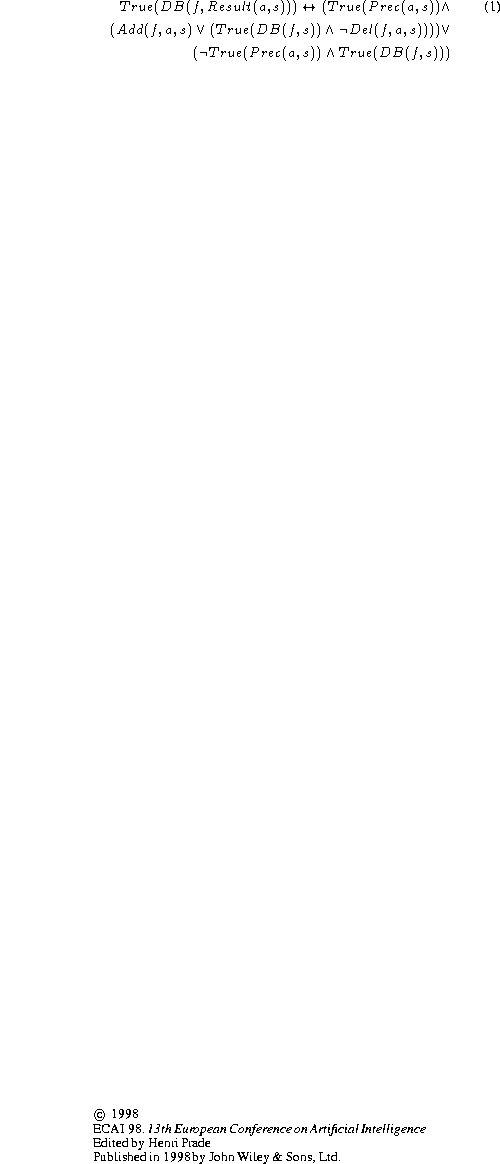Next: Blocks World Up: A Declarative Formalization of Previous: INTRODUCTION

# THE REPRESENTATION OF PLANNING PROBLEMS

The main ideas and axioms of the formalization presented in this section are taken from . STRIPS is a planning system that uses a database of logical formulasto represent information about a state. Associated with each situation s is a database of logical formulas describing what holds at that situation. The wffis true if formula f is in the database associated with situation s.

Each action a has a precondition, an add list and a delete list, which are formally characterized by the following atomic formulas: (1) True(Prec(a,s)) is true provided action a can be performed in situation s; (2) Del(f,a,s) is true if formula f is to be deleted when action a is performed in situation s; (3) Add(f,a,s) is true if formula f is to be added when action a is performed in situation s.

The function Result maps a situation s and an action a into the situation that results when action a is performed in situation s. The following axiom determines what formulas are in the database associated with the situation Result(a,s). If the precondition of action a is met at situation s, then the sentences on the delete list of a are deleted from the database, and the sentences on the add list of a are added to it.Josefina Sierra
Tue Jul 21 09:26:01 PDT 1998